Thank you for visiting nature.com. You are using a browser version with limited support for CSS. To obtain the best experience, we recommend you use a more up to date browser (or turn off compatibility mode in Internet Explorer). In the meantime, to ensure continued support, we are displaying the site without styles and JavaScript.

# Observation of the Nernst signal generated by fluctuating Cooper pairs

## Abstract

Long-range order is destroyed in a superconductor warmed above its critical temperature (Tc). However, amplitude fluctuations of the superconducting order parameter survive1 and lead to a number of well-established phenomena, such as paraconductivity2: an excess of charge conductivity due to the presence of short-lived Cooper pairs in the normal state. According to theory3, these pairs generate a transverse thermoelectric (Nernst) signal. In two dimensions, the magnitude of the expected signal depends only on universal constants and the superconducting coherence length, so the theory can be rigorously tested. Here, we present measurements of amorphous superconducting films of Nb0.15Si0.85. In this dirty superconductor, the lifetime of Cooper pairs exceeds the elastic scattering lifetime of quasiparticles in a wide temperature range above Tc and, consequently, their Nernst response dominates that generated by the normal electrons. We resolved a Nernst signal, which persists deep inside the normal state. Its amplitude is in excellent agreement with the theoretical prediction. This result provides an unambiguous case for a Nernst effect produced by short-lived Cooper pairs.

## Main

The Nernst effect, the generation of a transverse electric field by a longitudinal thermal gradient, has attracted considerable attention since the observation of a puzzling Nernst signal in the normal state of high-Tc cuprates4,5,6,7,8,9,10. In the context of the debate on the origin of this signal, Ussishkin, Sondhi and Huse (USH) calculated the contribution of amplitude fluctuations of the superconducting order parameter on thermoelectric transport3 and concluded that these fluctuations, described in the gaussian approximation and responsible for the well-established phenomenon of paraconductivity2 in the normal state of superconductors, should also generate a Nernst signal. In their model, the main contribution to the Nernst signal comes from the Aslamazov–Larkin term, which represents Cooper pairs with a finite lifetime above Tc (ref. 1). This lifetime decreases with increasing temperature. Therefore, in the presence of a thermal gradient, the pairs diffusing towards low temperature live longer than those diffusing towards high temperature, and thus the temperature gradient induces a net drift of pairs towards low temperature. The deflection of this current by a magnetic field produces a transverse voltage and hence a Nernst effect.

According to the USH calculations, the contribution of gaussian superconducting fluctuations to thermoelectricity leads to a finite off-diagonal component in the Peltier conductivity tensor, αx y, which is the ratio of the longitudinal charge current to the transverse thermal gradient (). In particular, in two dimensions, and for low magnetic fields Bφ0/2πξ2, where ξ is the coherence length and φ0 is the flux quantum, αx y is expected to follow this simple expression:Here, ℓB=(ħ/e B)1/2 is the magnetic length scale. Note that in equation (1), the three universal constants (Planck, Boltzmann and the charge of electron) combine to generate the quantum of thermoelectric conductance (kBe/h=3.3 nA K−1), a less celebrated concept compared with the quanta of electric1 (e2/h) or thermal112kB2T/3h) conductance. However, in the notation used by USH (as is often the case for theoretical papers), kB is taken as equal to unity and the quantum of thermoelectric conductance does not appear explicitly. As the magnitude of the coherence length, ξ, is the only parameter in equation (1), this theory is particularly apt for an unambiguous confrontation with experiment.

We have tested this theory by measuring the Nernst coefficient in amorphous films of NbxSi1−x (refs 1214), which exhibit the widely-documented features of superconductor–insulator transition in dirty two-dimensional superconductors15. The competition between superconducting and insulating ground states is controlled by the Nb concentration, x, the thickness, d, or the magnetic field16.

The data for the two samples shown in Figs 1 and 2 show that a finite measurable Nernst signal persists well above Tc. Before comparing this observation with the theoretical prediction by USH, let us argue that neither the excitations of the normal state, nor the superconducting phase fluctuations could be a plausible source for the observed Nernst signal. A rough scale for the normal-state Nernst signal is the product of the Seebeck coefficient (S) and the Hall angle (tanθ=RH/ρx x, where RH is the Hall coefficient and ρx x is the longitudinal resistivity). As seen in Fig. 2, in the entire range of our measurements, the Nernst coefficient, ν, is three orders of magnitude larger than Stanθ. In a multi-band metal, the contribution of carriers with different signs to Stanθ cancel out and its overall value could become smaller than ν (ref. 17), but such a possibility can be easily ruled out here. The hypothetical existence of two very small Fermi surface pockets hosting carriers of opposite sign with long mean-free-path seems implausible. The small value of tanθ≈2×10−5 simply reflects an extremely short electronic mean-free-path (of the order of interatomic distance 0.25 nm) and a conventional carrier density (the magnitude of RH=4.9×10−11 m3 C−1 is comparable to that reported18 for bulk Nb).

Could this signal be caused by phase fluctuations of the superconducting order parameter? This is also unlikely. In contrast to the underdoped cuprates, the carrier density in Nb0.15Si0.85 is comparable to any conventional metal. As the ‘phase stiffness’ of a superconductor is determined by its superfluid density19, there is no reason to speculate on the presence of preformed Cooper pairs without phase coherence in a wide temperature window above Tc as has been the case in the pseudogap state of the cuprates. In contrast to granular superconductors20, decreasing the thickness leads to a shift of the sharp superconducting transition and does not reveal a temperature scale other than the mean-field BCS (Bardeen–Cooper–Schrieffer) critical temperature. The variation of Tc with thickness has been attributed to the enhancement of the Coulomb interactions with the increase in the sheet resistance, Rsquare (ref. 21).

On the other hand, there is no reason to doubt the presence of amplitude fluctuations of the superconducting order parameter invoked by the USH theory. Now, the theory makes a precise prediction on the magnitude of αx y, but what is directly measured by the experiment is the Nernst coefficient, ν, which is intimately related to it. When the Hall angle is small and the contribution of superconducting fluctuations to charge conductivity is also small, there is a simple relationship between αx y, ν and the sheet resistance Rsquare: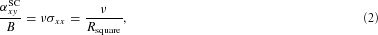where σ is the electric conductivity.

The validity of both conditions was checked in our experiment: tanθ≈2×10−5 and σSC=(e2/16ħ)(Tc/(TTc)) (ref. 1) is a few per cent of σx x when T>1.1Tc.

Because αx ySCB, it follows from relation (2) that ν should be independent of the magnetic field, in the low magnetic field region considered by this model. The data show that this is indeed the case. Therefore, we can directly determine αx y/B for each temperature in the zero-field limit using equation (2) and the data for ν and Rsquare. Now, equation (1) can be rewritten as: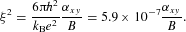The value of ξ obtained in this way for the two samples is shown in Fig. 3 as a function of the reduced temperature ɛ=(TTc)/Tc and allows a direct verification of the theory. Theoretically, the coherence length, ξ, should vary as ɛ−1/2 (ref. 1). Moreover, its absolute magnitude is expected to scale inversely with, which is in conformity with the ξ value found here: the ratio of the coherence lengths ξ1 and ξ2 for samples 1 and 2 is ξ1(ɛ=1)/ξ2(ɛ=1)=1.48, and the ratio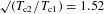. More quantitatively, the coherence length of a two-dimensional dirty superconductor is1: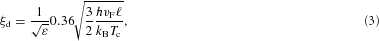where vF and ℓ are the Fermi velocity and the electronic mean-free-path. The most direct way to estimate vFℓ is to use the known values of the electric conductivity, σ≈6.4×104 Ω−1 cm−1 and the electronic specific heat, γe≈108 J K−1 m−3 (see the Methods section). The generic relationship between the specific heat and the thermal conductivity (κ), combined with the Wiedemann–Franz law yields: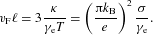This enables us to directly estimate vFℓ=4.35×10−5 m2 s−1 and, using equation (3), plot ξd(ɛ). As seen in Fig. 3, for both samples, for small values of ɛ, there is an excellent agreement between these two estimations of the coherence length. As the temperature rises,decreases faster than ξd. This discrepancy is not surprising because the ɛ−1/2 dependence of the coherence length ξd(ɛ) and the USH theory are valid only for small (<1) values of ɛ. Moreover, ξ becomes much smaller than the film thickness and the 2D limit is no longer valid. It is remarkable, however, that even for ɛ=10, the two values obtained for ξ differ by a mere factor of two.

In retrospect, it is not surprising that this effect is unambiguously observed for the first time in a dirty superconductor. According to the theory, what is universal is the magnitude of αx ySC, the expected Nernst signal is therefore larger when the normal-state conductivity is lower. Moreover, owing to the short mean-free-path of electrons, the normal-state Nernst effect becomes negligible, making the detection of the signal produced by superconducting fluctuations easier. On a more fundamental level, in a dirty superconductor, the lifetime of a fluctuating Cooper pair (τGLξ2/vFℓ (ref. 1)) exceeds the elastic lifetime of a normal electron (τel≈ℓ/vF) in a wide temperature window above Tc, paving the way for a dominant contribution of the gaussian fluctuations to the Nernst signal.

Charge conductivity, even in the presence of gaussian fluctuations, is dominated by the contribution of normal electrons. As we saw above, this is not the case for the Nernst effect, which (owing to the smallness of the normal-state Nernst effect) can be totally dominated by these fluctuations. This makes the Nernst effect a powerful probe of superconducting fluctuations.

We conclude by considering the field dependence of the Nernst coefficient. The USH calculation has been carried out for weak fields (ξB) and was only tested here in the zero-field limit. For T>Tc, as seen in Fig. 1, the Nernst signal reveals a field scale in its field dependence that increases with increasing temperature. This seems to simply reflect the decrease in the field scale associated with ξ(B*=ħ/(e ξ2)). For both samples, the Nernst signal does not vanish even with the application of a field as large as 4 T. This field is larger than all three field scales that can be associated with the destruction of superconductivity. These are (1) the critical field for the superconductor–insulator transition defined as the crossing field of R(B) curves at low temperatures, BSI (ref. 16); (2) the Pauli limit BP=1.84Tc (ref. 22); and (3) the orbital limit Borb=φ0/2πξ2. The values for both samples are shown in Table 1, where it seems that the upper critical field Bc2 is set by the Pauli limit, that is, Bc2=BSIBP<Borb. It is natural to assume that the superconducting long-range order is indeed destroyed at Bc2, but the superconducting fluctuations persist and gradually fade away above Bc2, as they do above Tc. The contribution of the gaussian fluctuations to the Nernst effect in high magnetic fields remains a challenging question for both theory and experiment.

## Methods

The two amorphous thin films of Nb0.15Si0.85 used in this study were prepared as described elsewhere12,13. The nominal concentration of Nb in the two samples used in this study was the same (x=0.15) and the difference in Tcs, (0.165 K in sample 1 with d=12.5 nm and 0.38 K in sample 2 with d=35 nm), is mainly due to the difference in thickness of the two samples. The critical temperature was defined as the mid-height of the resistive transition at zero field. A setup with one resistive heater, two RuO2 thermometers and two lateral contacts23 was used to measure the thermoelectric and the electric coefficients of each sample in a dilution cryostat. At T0.19 K, we could resolve a d.c. voltage of 1 nV and a temperature difference of 0.1 mK. The magnitude of the electronic specific heat used for the estimation of the coherence length is based on the magnitude of γe in bulk Nb (ref. 24) and the concentration of itinerant electrons provided by the Nb fraction, as confirmed by direct measurements of specific heat in NbxSi1−x thin films of a lower concentration13.

## References

1. Larkin, A. & Varlamov, A. Theory of Fluctuations in Superconductors (Clarendon, Oxford, 2005).

2. Glover, R. E. Ideal resistive transition of a superconductor. Phys. Lett. A 25, 542–544 (1967).

3. Ussishkin, I., Sondhi, S. L. & Huse, D. A. Gaussian superconducting fluctuations, thermal transport, and the Nernst effect. Phys. Rev. Lett. 89, 287001 (2002).

4. Xu, Z. A. et al. Vortex-like excitations and the onset of superconducting phase fluctuation in underdoped La2−xSrxCuO4 . Nature 406, 486–488 (2000).

5. Wang, Y. et al. Onset of the vortex-like Nernst signal above Tc in La2−xSrxCuO4 and Bi2Sr2−yLayCuO6 . Phys. Rev. B 64, 224519 (2001).

6. Wang, Y. et al. Dependence of upper critical field and pairing strength on doping in cuprates. Science 299, 86–89 (2003).

7. Capan, C. et al. Entropy of vortex cores near the superconductor-insulator transition in an underdoped cuprate. Phys. Rev. Lett. 88, 056601 (2002).

8. Wen, H. H. et al. Two-dimensional feature of the Nernst effect in normal state of underdoped La2−xSrxCuO4 single crystals. Europhys. Lett. 63, 583–589 (2003).

9. Rullier-Albenque, F. et al. Nernst effect and disorder in the normal state of high-Tc cuprates. Phys. Rev. Lett. 96, 067002 (2006).

10. Wang, Y., Li, L. & Ong, N. P. Nernst effect in high-Tc superconductors. Phys. Rev. B 73, 024510 (2006).

11. Schwab, K., Henriksen, E. A., Worlock, J. M. & Roukes, M. L. Measurement of the quantum of thermal conductance. Nature 404, 974–977 (2000).

12. Dumoulin, L., Bergé, L., Lesueur, J., Bernas, H. & Chapellier, M. Nb-Si thin films as thermometers for low temperature bolometers. J. Low Temp. Phys. 93, 301–306 (1993).

13. Marnieros, S., Bergé, L., Juillard, A. & Dumoulin, L. Dynamical properties near the metal-insulator transition: evidence for electron-assisted variable range hopping. Phys. Rev. Lett. 84, 2469–2472 (2000).

14. Lee, H.-L., Carini, J. P., Baxter, D. V., Henderson, W. & Grüner, G. Quantum-critical conductivity scaling for a metal-insulator transition. Science 287, 633–636 (2000).

15. Goldman, A. M. & Markovic, N. Superconductor–insulator transitions in the two-dimensional limit. Phys. Today 51, 39–44 (1998).

16. Aubin, H. et al. Magnetic-field-induced quantum superconductor-insulator transition in Nb0.15Si0.85 . Phys. Rev. B 73, 094521 (2006).

17. Bel, R., Behnia, K. & Burger, H. Ambipolar Nernst effect in NbSe2 . Phys. Rev. Lett. 91, 066602 (2003).

18. Gilchrist, J. le G. & Vallier, J.-C. Hall effect in superconducting niobium and alloys. Phys. Rev. B 3, 3878–3886 (1971).

19. Emery, V. J. & Kivelson, S. A. Importance of phase fluctuations in superconductors with small superfluid density. Nature 374, 434–437 (1995).

20. Jaeger, H. M., Haviland, D. B., Orr, B. G. & Goldman, A. M. Onset of superconductivity in ultrathin granular metal-films. Phys. Rev. B 40, 182–196 (1989).

21. Maekawa, S., Ebisawa, H. & Fukuyama, H. Upper critical field in two-dimensional superconductors. J. Phys. Soc. Jpn 52, 1352–1360 (1983).

22. Clogston, A. M. Upper limit for the critical field in hard superconductors. Phys. Rev. Lett. 9, 266–267 (1962).

23. Bel, R. et al. Giant Nernst effect in CeCoIn5 . Phys. Rev. Lett. 92, 217002 (2004).

24. Leupold, H. A. & Boorse, H. A. Superconducting and normal specific heats of a single crystal of niobium. Phys. Rev. 134, A1322–A1328 (1964).

## Acknowledgements

This work is partially supported by Agence Nationale de la Recherche. We are grateful to C. Capan, A. Kapitulnik, M. Grilli, D. Huse, S. Kivelson, S. Sondhi and I. Ussishkin for useful discussions.

## Author information

Authors

### Contributions

C.A.M.-K., L.B. and L.D. prepared the samples. A.P. (assisted by H.A. and K.B.) carried out the measurements. H.A. and K.B. (discussing with A.P. and J.L.) interpreted and analysed the data. K.B. wrote the text.

### Corresponding authors

Correspondence to H. Aubin or K. Behnia.

## Ethics declarations

### Competing interests

The authors declare no competing financial interests.

## Rights and permissions

Reprints and Permissions

Pourret, A., Aubin, H., Lesueur, J. et al. Observation of the Nernst signal generated by fluctuating Cooper pairs. Nature Phys 2, 683–686 (2006). https://doi.org/10.1038/nphys413

• Accepted:

• Published:

• Issue Date:

• DOI: https://doi.org/10.1038/nphys413

• ### Upper critical field and superconductor-metal transition in ultrathin niobium films

• Iryna Zaytseva
• Aleksander Abaloszew
• Marta Z. Cieplak

Scientific Reports (2020)

• ### Reentrant Resistive Behavior and Dimensional Crossover in Disordered Superconducting TiN Films

• Svetlana V. Postolova
• Alexey Yu. Mironov
• Tatyana I. Baturina

Scientific Reports (2017)

• ### Separating pairing from quantum phase coherence dynamics above the superconducting transition by femtosecond spectroscopy

• T. Kurosawa
• D. Mihailovic

Scientific Reports (2015)

• ### Colossal thermomagnetic response in the exotic superconductor URu2Si2

• T. Yamashita
• Y. Shimoyama
• Y. Matsuda

Nature Physics (2015)

• ### Superconducting fluctuations in organic molecular metals enhanced by Mott criticality

• Moon-Sun Nam
• Cécile Mézière
• Arzhang Ardavan

Scientific Reports (2013)

## Search